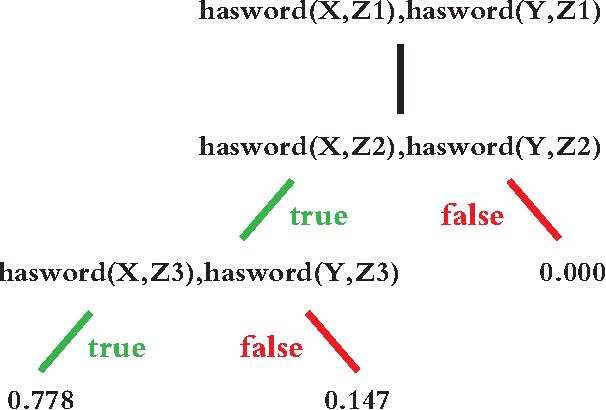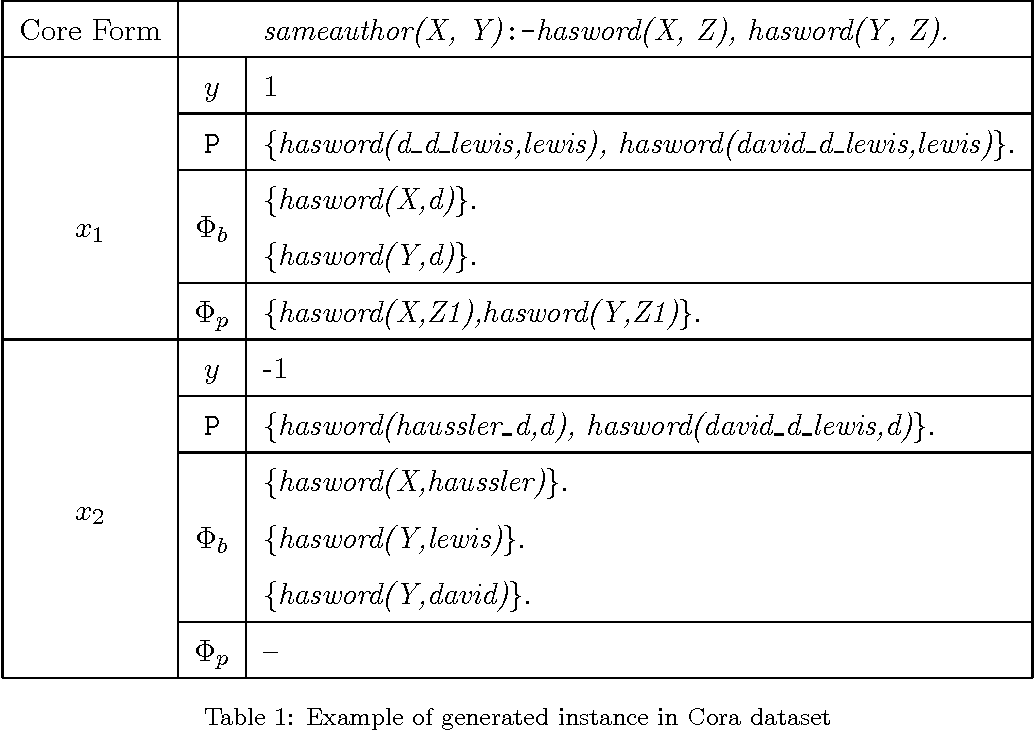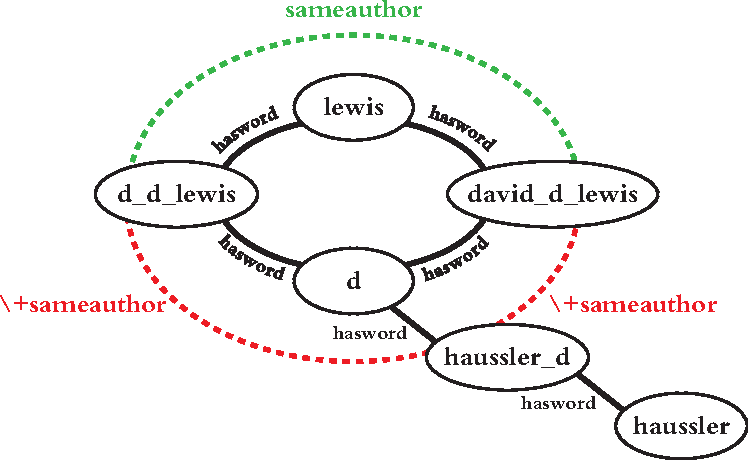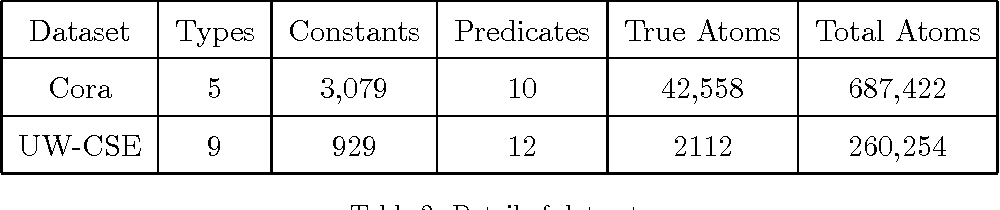Recent years have seen a surge of interest in Probabilistic Logic Programming (PLP) and Statistical Relational Learning (SRL) models that combine logic with probabilities. Structure learning of these systems is an intersection area of Inductive Logic Programming (ILP) and statistical learning (SL). However, ILP cannot deal with probabilities, SL cannot model relational hypothesis. The biggest challenge of integrating these two machine learning frameworks is how to estimate the probability of a logic clause only from the observation of grounded logic atoms. Many current methods models a joint probability by representing clause as graphical model and literals as vertices in it. This model is still too complicate and only can be approximate by pseudo-likelihood. We propose Inductive Logic Boosting framework to transform the relational dataset into a feature-based dataset, induces logic rules by boosting Problog Rule Trees and relaxes the independence constraint of pseudo-likelihood. Experimental evaluation on benchmark datasets demonstrates that the AUC-PR and AUC-ROC value of ILP learned rules are higher than current state-of-the-art SRL methods.

* 19 pages, 2 figuresClick To Get Code For Paper